# 4. Content Markup

## 4.4 The Content Markup Elements

This section provides detailed descriptions of the MathML content tags. They are grouped in categories which broadly reflect the area of mathematics from which they come, and also the grouping in the MathML DTD. There is no linguistic difference in MathML between operators and functions. Their separation here and in the DTD is for reasons of clarity.

When working with the content elements, keep in mind the following.

• The role of the content elements is analogous to data entry in a mathematical system. The information that is provided is there to facilitate the successful parsing of an expression as the intended mathematical object by a receiving application.

• MathML content elements do not by themselves "perform" any mathematical evaluations or operations. They do not "evaluate" in a browser and any "action" that is ultimately taken on those objects is determined entirely by the receiving mathematical application. For example, editing programs and applications geared to computation for the lower grades would typically leave 3 + 4  as is, while computational systems targeting a more advanced audience might evaluate this to as 7. Similarly, some computational systems might evaluate sin(0) to 0 while others would leave it unevaluated. Yet other computational systems might be unable to deal with pure symbolic expressions sin(x) and may even regard it as a data entry error. None of this has any bearing on the correctness of the original MathML representation. Where evaluation is mentioned at all in the descriptions below, it is merely to help clarify the meaning of the underlying operation.

• Apart from the instances where there is an explicit interaction with presentation tagging, there is no required rendering (visual or aural) -- only a suggested default. As such, the presentations that are included in this section are merely to help communicate to the reader the intended mathematical meaning by association with the same expression written in a more traditional notation.

The available content elements are:

### 4.4.1 Token Elements

#### 4.4.1.1 <cn>

##### Discussion
The cn element is used to specify actual numerical constants. The content model must provide sufficient information that a number may be entered as data into a computational system. By default, it represents a signed real number in base 10. Thus, the content normally consists of PCDATA restricted to a sign, a string of decimal digits and possibly a decimal point, or alternatively one of the predefined symbolic constants such as &pi;.

The cn element uses the attribute type to represent other types of numbers such as integer, rational, real, complex etc. and base to specify the numerical base.

In addition to simple PCDATA, cn accepts as content PCDATA separated by the <sep/> element. This determines the different parts needed to construct a rational or complex-cartesian number.

Alternative input notations for numbers are possible, but must be explicitly defined by using the definitionURL attribute to refer to a written specification of how a <sep/> separated sequence of real numbers is to be interpreted.

##### Attributes

All attributes are CDATA:

• type: real | integer | rational | complex-cartesian | complex-polar | constant
• base: number (CDATA for XML DTD) between 2 and 36.
• definitionURL: URL pointing to an alternative definition.
##### Examples:
<cn type="real"> 12345.7 </cn>
<cn type="integer"> 12345 </cn>
<cn type="integer" base="16"> AB3 </cn>
<cn type="rational"> 12342 <sep/> 2342342 </cn>
<cn type="complex-cartesian"> 12.3 <sep/> 5 </cn>
<cn type="complex-polar"> 2 <sep/> 3.1415 </cn>
<cn type="constant"> &pi; </cn>
##### Default Renderings

By default, contiguous blocks of PCDATA contained in cn elements should render as if it were wrapped in an mn presentation elements. Similarly, presentation markup contained in a cn element should render as it normally would. A mixture of PCDATA and presentation markup should render as if it were contained wrapped in an mrow element, with contiguous blocks of PCDATA wrapped in mn elements.

However, not all mathematical systems that encounter content based tagging do visual or aural rendering. The receiving applications are free to make use of a number in the manner it normally handles numerical data. Some systems might simplify the rational number 12342/2342342 to 6171/1171171 while pure floating point based systems might approximate this as .5269085385e-2. All numbers might be re-expressed in base 10. The role of MathML is simply to record enough information about the mathematical object and its structure so that it may be properly parsed.

The following renderings of the above MathML expressions are included both to help clarify the meaning of the corresponding MathML encoding and as suggestions for authors of rendering applications. In each case, no mathematical evaluation is intended or implied.

12345.7
12345
AB316
12342 / 2342342
12.3 + 5 i
Polar( 2 , 3.1415 )
&pi;

#### 4.4.1.2 <ci>

##### Discussion

The ci element is used to specify a symbolic name. Such names are used to identify types of mathematical objects. By default they are assumed to represent complex scalars. The ci element may contain arbitrary presentation markup in its content (see chapter 3) so that its presentation as a symbol can be very elaborate.

The ci element uses the type attribute to specify the type of object that it represents. Valid types include integer, rational, real, float, complex, complex-polar, complex-cartesian, constant, and more generally, any of the names of the MathML container elements (e.g. vector) or their type values. The definitionURL attribute can be used to extend the definition of ci to include other types. For example, a more advanced use might require a "complex-vector".

##### Examples
1. <ci> x </ci>
2. <ci type="vector"> V </ci>
3. <ci>
<msub>
<mi>x</mi>
<mi>a</mi>
</msub>
</ci>

##### Default Rendering
If the content of a ci element is tagged using presentation tags, that presentation is used. If no such tagging is supplied then the PCDATA content would typically be rendered as if it were the content of an mi element. A renderer may wish to make use of the value of the type attribute to improve on this. For example, a symbol of type vector might be rendered using a bold face. Typical renderings of the above symbols are:
1. x
2. V
3. xi

### 4.4.2 Basic Content Elements

#### 4.4.2.1 <apply>

##### Discussion

The apply element allows a function or operator to be applied to its arguments. Nearly all expression construction in MathML content markup is carried out by applying operators or functions to arguments. The first child of apply is the operator, to be applied, with the other child elements as arguments.

The apply element is conceptually necessary in order to distinguish between a function or operator, and an instance of its use. The expression constructed by applying a function to 0 or more arguments is always an element from the range of the function.

Proper usage depends on the operator that is being applied. For example, the plus operator may have zero or more arguments. while the minus operator requires 1 or 2 arguments to be properly formed.

If the object being applied as a function is not already one of the elements known to be a function (such as fn, sin or plus) then it is treated as if it were the contents of an fn element.

Some operators such as diff and int make use of "named" arguments. These special arguments are elements which appear as children of the apply element and identify "parameters" such as the variable of differentiation or the domain of integration.

##### Examples
1. <apply><factorial/>
<cn>3</cn>
</apply>
2. <apply><plus/>
<cn>3</cn>
<cn>4</cn>
</apply>
3. <apply><sin/>
<ci>x</ci>
</apply>

##### Default Rendering

A mathematical system which has been passed an apply element is free to do with it whatever it normally does with such mathematical data. It may be that no rendering is involved (e.g. a syntax validator), or that the "function application" is evaluated and that only the result is rendered (e.g. sin(0) -> 0).

When an unevaluated "function application" is rendered there are a wide variety of appropriate renderings. The choice often depends on the function or operator being applied. Applications of basic operations such as plus are generally presented using an infix notation while applications of sin would use a more traditional functional notation such as sin (x). Consult the default rendering for the operator being applied.

Applications of user-defined functions (see fn) which are not evaluated by the receiving or rendering application would typically render using a traditional functional notation unless an alternative presentation is specified using the semantics tag.

#### 4.4.2.2 <reln>

##### Discussion
The reln element is used to construct an equation or relation. Relations are constructed in a manner exactly analogous to the use of apply. A separate construct is used because the manner in which relations are used differs substantially from that of general algebraic expressions.

The first child of reln is the relational operator, to be applied, with the other child elements as arguments.

See the Usage Guide for further details.

##### Examples and Usage
<reln><eq/>
<ci> a </ci>
<ci> b </ci>
</reln>

<reln><lt/>
<ci> a </ci>
<ci> b </ci>
</reln>

a = b
a < b

#### 4.4.2.3 <fn>

##### Discussion
The fn element makes explicit the fact that a more general MathML object is intended to be used in the same manner as if it were a pre-defined function such as sin or plus.

It is the primary mechanism used to extend the collection of "known" mathematical functions. The definitionURL attribute allows for a specific written definition to be referenced by URL. (New functions may also be introduced by using a declaration in conjunction with a lambda expression.)

##### Examples
1. <fn><ci> L </ci> </fn>

2. <fn definitionURL="http://www.openmath.org/CDs/MyDC/MyDefinition">
<ci>
<msub>
<mi>J</mi>
<mn>0</mn>
</msub>
</ci>
</fn>

3. <fn>
<apply> <plus/>
<ci> f </ci>
<ci> g </ci>
</apply>
</fn>

##### Default Rendering
An fn object is rendered in the same way as its content.
1. L
2. J0
3. f + g

#### 4.4.2.4 <interval>

##### Discussion

The interval element is used to represent simple mathematical intervals of the real number line. It takes an attribute closure which can take on any of the values open, closed, open-closed, or closed-open, with a default value of closed.

More general domains are constructed by using the condition and bvar elements to bind free variables to constraints.

The interval element expects either two child elements which evaluate to real numbers or one child element which is a condition defining the interval. interval accepts a closure attribute which specifies if the interval is open, closed, or half open.

##### Examples
1. <interval>
<ci> a </ci>
<ci> b </ci>
</interval>

2. <interval closure="open-closed">
<ci> a </ci>
<ci> b </ci>
</interval>

[a,b]
(a,b]

#### 4.4.2.5 <inverse/>

##### Discussion

The inverse element is applied to a function in order to construct a generic expression for the functional inverse of that function. (See also the discussion of inverse in section 4.1.3.7). As with other MathML functions, inverse may either be applied to arguments, or it may appear alone, in which case it represents an abstract inversion operator acting on other functions.

A typical use of the inverse element is in an HTML document discussing a number of alternative definitions for a particular function so that there is a need to write and define f (-1)(x).

To associate a particular definition with f (-1), use the definitionURL attribute.

##### Examples
1. <apply><inverse/>
<ci> f </ci>
</apply>

2. <apply><inverse definitionURL="MyDefinition"/>
<ci> f </ci>
</apply>

3. <apply>
<apply><inverse/>
<ci type="matrix"> a </ci>
</apply>
<ci> A </ci>
</apply>

##### Default Rendering
The default rendering for a functional inverse makes use of a parenthesized exponent as in f (-1)(x).

#### 4.4.2.6 <sep/>

##### Discussion
The sep element is to separate PCDATA into separate tokens for parsing the contents of the various specialized forms of the cn elements. For example sep is used when specifying the real and imaginary parts of a complex number (see Section 4.4.1). If it occurs between proper elements, it is a MathML error.
##### Examples
<cn type="complex"> 3 <sep/> 4 </cn>

##### Default Rendering
The sep element is not directly rendered. (see Section 4.4.1 cn)

#### 4.4.2.7 <condition>

##### Discussion
The condition element is used to place a condition on one or more free variables or identifiers. The conditions may be specified in terms of relations that are to be satisfied by the variables, including general relationships such as set membership.

It is used to define general sets and lists in situations where the elements cannot be explicitly enumerated. Condition contains either a single reln or apply element; the apply element is used to construct compound conditions." For example, it is used below to describe the set of all x such that x < 5. See the discussion on sets in section section 4.4.6.

See the Usage Guide for further details.

##### Examples
1.  <condition>
<reln><in/><ci> x </ci><ci type="set"> R </ci></reln>
</condition>

2.  <condition>
<apply> <and/>
<reln><gt/><ci> x </ci><cn> 0 </cn></reln>
<reln><lt/><ci> x </ci><cn> 1 </cn></reln>
</apply>
</condition>

3.  <apply>
<max/>
<bvar><ci> x </ci></bvar>
<condition>
<apply> <and/>
<reln><gt/><ci> x </ci><cn> 0 </cn></reln>
<reln><lt/><ci> x </ci><cn> 1 </cn></reln>
</apply>
</condition>
<apply>
<minus/>
<ci> x </ci>
<apply>
<sin/>
<ci> x </ci>
</apply>
</apply>
</apply>

##### Default Rendering
1. x in R
2. x > 0 and x < 1
3. maxx { x - sin x | 0 < x < 1}

#### 4.4.2.8 <declare>

##### Discussion
The declare construct has two primary roles. The first is to change or set the default attribute values for a specific mathematical object. The second is to establish an association between a "name" and an object. Once a declaration is in effect, the "name" object acquires the new attribute settings, and (if the second object is present) all the properties of the associated object.

The various attributes of the declare element assign properties to the object being declared or determine where the declaration is in effect.

By default, the scope of a declaration is "local" to the surrounding container element. Setting the value of the scope attribute to "global" extends the scope of the declaration to the enclosing math element. As discussed in Section 4.3.2.8, MathML contains no provision for making document-wide declarations at present, though it is anticipated this capability will be added in future revisions of MathML, when supporting technologies become available. declare takes 1 or 2 children. The first, mandatory, child is a ci containing the identifier being declared.

<declare type="vector"> <ci> V </ci> </declare>

The second, optional, child is a constructor initialising the variable
<declare type="vector">
<ci> V </ci>
<vector>
<cn> 1 </cn><cn> 2 </cn><cn> 3 </cn>
</vector>
</declare>


The constructor type and the type of the element declared must agree. For example, if the type attribute of the declaration is fn, the second child (constructor) must be an element equivalent to an fn element (This would include actual fn elements, lambda elements and any of the defined function in the basic set of content tags.) If no type is specified in the declaration then the type attribute of the declared name is set to the type of the constructor (second child) of the declaration. The type attribute of the declaration can be especially useful in the special case of the second element being a symantic tag,

##### Attributes

All attributes are CDATA:

• type - defines the MathML element type of the identifier declared.
• scope - defines the scope of application of the declaration.
• nargs - number of arguments for function declarations.
• occurrence - describes operator usage as "prefix", "infix" or "function-model" indications
• definitionURL - URL pointing to detailed semantics of the function.
##### Examples
The declaration

<declare type="fn" nargs="2" scope="local">
<ci> f </ci>
<apply><plus/>
<ci> F </ci><ci> G </ci>
</apply>
</declare>

declares f to be a two-variable function with the property that f(x,y) = (F + G)(x,y).

The declaration

<declare type="fn">
<ci> J </ci>
<lambda>
<bvar><ci> x </ci></bvar>
<apply><ln/>
<ci> x </ci>
</apply>
</lambda>
</declare>

associates the name J with a one-variable function defined so that J(x) = ln x (Note that because of the type attribute of the declare element, the second argument must be something of type fn, namely a known function like sin, an fn construct, or a lambda construct.)

The type attribute on the declaration is only necessary if if the type cannot be inferred from the type of the second argument.

Even when a declaration is in effect it is still possible to override attributes values selectively as in <ci type="integer"> V </ci> . This capability is needed in order to write statements of the form "Let S be a member of S".

##### Default Rendering

Since the declare construct is not directly rendered, most declarations are likely to be invisible to a reader. However, declarations can produce quite different effects in an application which evaluates or manipulates MathML content. While the declaration

<declare>
<ci> v </ci>
<vector>
<cn> 1 </cn>
<cn> 2 </cn>
<cn> 3 </cn>
</vector>
</declare>

is active the symbol v acquires all the properties of the vector, and even its dimension and components have meaningful values. This may affect how v is rendered by some applications, as well as how it is treated mathematically.

#### 4.4.2.9 <lambda>

##### Discussion
The lambda element is used to construct a user-defined function from an expression and one or more free variables. The lambda construct with n internal variables takes n + 1 children. The first n children identify the variables which are used as placeholders in the last child for actual parameter values.

See the Usage Guide for further details.

##### Examples
The following markup represents lambda(x, sin x+1).

<lambda>
<bvar><ci> x </ci></bvar>
<apply><sin/>
<apply><plus/>
<ci> x </ci>
<cn> 1 </cn>
</apply>
</apply>
</lambda>



The following examples constructs a one argument function in which the argument b specifies the upper bound of a specific definite integral.

<lambda>
<bvar><ci> b </ci></bvar>
<apply>
<int/>
<bvar>
<ci> x </ci>
</bvar>
<lowlimit>
<ci> a </ci>
</lowlimit>
<uplimit>
<ci> b </ci>
</uplimit>
<apply><fn><ci> f </ci></fn>
<ci> x </ci>
</apply>
</apply>
</lambda>

Such constructs are often used conjunction with declare to construct new functions.
Lambda(x,f(x))

#### 4.4.2.10 <compose/>

##### Discussion
The <compose/> element represents the function composition operator. Note that MathML makes no assumption about the domain and range of the constituent functions in a composition; the domain of the resulting composition may be empty.

To override the default semantics for the <compose/> element, or to associate a more specific definition for function composition, use the definitionURL attribute.

See the Usage Guide for further details.

##### Examples
The following markup represents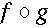.

<apply><compose/>
<fn><ci> f </ci></fn>
<fn><ci> g </ci></fn>
</apply>


The following markup represents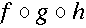.

<apply><compose/>
<ci type="fn"> f </ci>
<ci type="fn"> g </ci>
<ci type="fn"> h </ci>
</apply>



The following examples both represent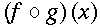.


<apply>
<apply><compose/>
<fn><ci> f </ci></fn>
<fn><ci> g </ci></fn>
</apply>
<ci> x </ci>
</apply>



<apply><fn><ci> f </ci></fn>
<apply>
<fn><ci> g </ci></fn>
<ci> x </ci>
</apply>
</apply>


##### Default Rendering#### 4.4.2.11<ident/>

##### Discussion

The indent element represents the identity function. MathML makes no assumption about the function space in which the identity function resides. That is, proper interpretation of the domain (and hence range) of the identity function depends on the context in which it is used.

To override the default semantics for the <ident/> element, or to associate a more specific definition, use the definitionURL attribute.

See the Usage Guide for further details.

##### Examples
The following markup encoded the expression.

<reln><eq/>
<apply><compose/>
<fn><ci> f </ci></fn>
<apply><inverse/>
<fn><ci> f </ci></fn>
</apply>
</apply>
<ident/>
</reln>


id

### 4.4.3 Arithmetic, Algebra and Logic

#### 4.4.3.1 <quotient/>

##### Discussion

The quotient element is the operator used for division modulo a particular base. When the quotient operator is applied to integer arguments a and b, the result is the "quotient of a divided by b". That is, quotient returns the unique integer, q such that a = q b + r. (In common usage, q is called the quotient and r is the remainder.)

The quotient element takes the attribute definitionURL if it is desired to override the default semantics.

The quotient element is a binary arithmetic operator.

See the Usage Guide for further details.

##### Example
<apply><quotient/>
<ci> a </ci>
<ci> b </ci>
</apply>

Various mathematical applications will use this data in different ways. Editing applications might choose an image such as shown below, while a computationally based application would evaluate it to 2 when a = 13 and b = 5.
##### Default Rendering
There is no commonly used notation for this concept. Some possible renderings are
1. quotient of a divided by b
2. integer part of a/b
3.#### 4.4.3.2 <exp/>

##### Discussion

The exp element represents the exponential function associated with the inverse of the ln function. In particular, exp(1) is approximately 2.718281828.

exp takes the attribute definitionURL which may be used to override the default semantics.

The exp element is a unary arithmetic operator.

See the Usage Guide for further details.

##### Example
<apply><exp/>
<ci> x </ci>
</apply>

ex

#### 4.4.3.3 <factorial/>

##### Discussion

The factorial element is used to construct factorials.

The factorial element takes the attribute definitionURL which may be used to override the default semantics.

The factorial element is a unary arithmetic operator.

See the Usage Guide for further details.

##### Example
<apply><factorial/>
<ci> n </ci>
</apply>

If this were evaluated at n = 5 it would evaluate to 120.
n!

#### 4.4.3.4 <divide/>

##### Discussion
The divide element is the division operator.

The divide element takes the attribute definitionURL which may be used to override the default semantics.

The divide element is a binary arithmetic operator. See the Usage Guide for further details.

##### Example
<apply> <divide/>
<ci> a </ci>
<ci> b </ci>
</apply>

As a MathML expresssion, this does not evaluate. However, on receiving such an expression, some applications may attempt to evaluate and simplify the value. For example, when a=5 and b=2 some mathematical applications may evaluate this to 2.5 while others will treat is as a rational number.
a/b

#### 4.4.3.5 <max/> and <min/>

##### Discussion

The elements max and min are used to compare the values of their arguments. They return the maximum and minimum of these values respectively.

The max and min elements take the attribute definitionURL which can be used to override the default semantics.

The max and min elements are n-ary arithmetic operators. See the Usage Guide for further details.

##### Examples
When the objects are to be compared explicitly they are listed as arguments to the function as in:
<apply><max/>
<ci> a </ci>
<ci> b </ci>
</apply>

The elements to be compared may also be described using bound variables with a condition element and an expression to be maximised, as in:
<apply><min/>
<bvar><ci>x</ci></bvar>
<condition>
<reln><notin/><ci> x </ci><ci type="set"> B </ci></reln>
</condition>
<apply>
<power/>
<ci> x </ci>
<cn> 2 </cn>
</apply>
</apply>

Note that the bound variable may be implicit:
<apply><max/>
<condition>
<apply><and/>
<reln><in/><ci>x</ci><ci type="set">B</ci></reln>
<reln><notin/><ci>x</ci><ci type="set">C</ci></reln>
</apply>
</condition>
</apply>

##### Default Rendering
1. max {a, b}
2. minx { x2 | x notin B }
3. max { x in B and x notin C}

#### 4.4.3.6 <minus/>

##### Discussion

The minus element is the subtraction operator.

The minus element takes the attribute definitionURL which can be used to override the default semantics.

The minus element can be used as a unary arithemtic operator (e.g. to represent -x) or as a binary arithmetic operator (e.g. to represent x-y). See the Usage Guide for further details.

##### Example
<apply> <minus/>
<ci> x </ci>
<ci> y </ci>
</apply>

If this were evaluated at x= 5 and y = 2 it would yield 3.
x - y

#### 4.4.3.7 <plus/>

##### Discussion
The plus element is the addition operator.

The plus element takes the attribute definitionURL which can be used to override the default semantics.

The plus element is an n-ary arithemtic operator. See the Usage Guide for further details.

##### Example
<apply><plus/>
<ci> x </ci>
<ci> y </ci>
<ci> z </ci>
</apply>

If this were evaluated at x = 5, y = 2 and z = 1 it would yield 8.
x + y + z

#### 4.4.3.8 <power/>

##### Discussion
The power element is generic exponentiation operator. That is, when applied to arguments a and b, it returns the value the "a to the power of b".

The power element takes the attribute definitionURL which can be used to override the default semantics.

The power element is an binary arithmetic operator. See the Usage Guide for further details.

##### Example
<apply><power/>
<ci> x </ci>
<cn> 3 </cn>
</apply>

If this were evaluated at x= 5 it would yield 125.
x3

#### 4.4.3.9 <rem/>

##### Discussion

The rem element is the operator which returns the "remainder" of a division modulo a particular base. When the rem operator is applied to integer arguments a and b, the result is the "remainder of a divided by b". That is, rem returns the unique integer, r such that a = q b + r, where r < q. (In common usage, q is called the quotient and r is the remainder.)

The rem element takes the attribute definitionURL which can be used to override the default semantics.

The rem element is a binary arithmetic operator. See the Usage Guide for further details.

##### Example
<apply><rem/>
<ci> a </ci>
<ci> b </ci>
</apply>

If this were evaluated at a = 15 and b = 8 it would yield 7.
a mod b

#### 4.4.3.10 <times/>

##### Discussion

The times element is the multiplication operator.

times takes the attribute definitionURL which can be used to override the default semantics.

##### Example
<apply> <times/>
<ci> a </ci>
<ci> b </ci>
</apply>

If this were evaluated at a = 5.5 and b = 3 it would yield 16.5.
a b

#### 4.4.3.11 <root/>

##### Discussion

The root element is used to construct roots. The kind of root to be taken is specified by a degree element, which should be given as the first child of the apply element enclosing the root element. Thus, square roots correspond to the case where degree contains the value 2, cube roots correspond to 3, and so on. If no degree is present, a default value of 2 is used.

The root element takes the attribute definitionURL which can be used to override the default semantics.

The root element is an operator taking qualifiers. See the Usage Guide for further details.

##### Example

The nth root of a is is given by

<apply> <root/>
<degree><ci> n </ci></degree>
<ci> a </ci>
</apply>

##### Default Rendering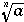#### 4.4.3.12 <gcd/>

##### Discussion

The gcd element is used to denote the greatest common divisor of its arguments.

The gcd takes the attribute definitionURL which can be used to override the default semantics.

The gcd element is an n-ary operator. See the Usage Guide for further details.

##### Example
<apply> <gcd/>
<ci> a </ci>
<ci> b </ci>
<ci> c </ci>
</apply>

If this were evaluated at a = 15, b = 21, c = 48 it would yield 3.
GCD(a, b, c)

#### 4.4.3.13 <and/>

##### Discussion

The and element is the boolean "and" operator.

The and element takes the attribute definitionURL which can be used to override the default semantics.

The and element is an n-ary logical operator. See the Usage Guide for further details.

##### Example
<apply><and/>
<ci> a </ci>
<ci> b </ci>
</apply>

If this were evaluated and both a and b had truth values of "true", then the value would be "true".
a and b

#### 4.4.3.14 <or/>

##### Discussion

The or element is the boolean "or" operator.

The or element takes the attribute definitionURL which can be used to override the default semantics.

The or element is an n-ary logical operator. See the Usage Guide for further details.

##### Example
<apply><or/>
<ci> a </ci>
<ci> b </ci>
</apply>

a or b

#### 4.4.3.15 <xor/>

##### Discussion

The xor element is the boolean "exclusive or" operator.

xor takes the attribute definitionURL which can be used to override the default semantics.

The xor element is an n-ary logical operator. See the Usage Guide for further details.

##### Example
<apply><xor/>
<ci> a </ci>
<ci> b </ci>
</apply>

a XOR b

#### 4.4.3.16 <not/>

The not operator is the boolean "not" operator.

The not element takes the attribute definitionURL which can be used to override the default semantics.

The not element is an unary logical operator. See the Usage Guide for further details.

##### Example
<apply><not/>
<ci> a </ci>
</apply>

NOT a

#### 4.4.3.17 <implies/>

##### Discussion

The implies element is the boolean "implies" relational operator.

The implies element takes the attribute definitionURL which can be used to override the default semantics.

The implies element is a binary logical operator. See the Usage Guide for further details.

##### Example
<reln><implies/>
<ci> A </ci>
<ci> B </ci>
</reln>

Mathematical applications designed for the evaluation of such expressions would evaluate this to "true" when a = "false" and b = "true".
##### Default Rendering#### 4.4.3.18 <forall/>

The forall element represents the universal quantifier of logic. It must used in conjunction with one or more bound variables, an optional condition element, and an assertion, which may either take the form of an apply or reln element.

The forall element takes the attribute definitionURL which can be used to override the default semantics.

The forall element is an quantifier. See the Usage Guide for further details.

##### Examples

The first example encodes the sense of the expression "for all x, x - x = 0".


<apply>
<forall/>
<bvar><ci> x </ci></bvar>
<reln><eq/>
<apply>
<minus/><ci> x </ci><ci> x </ci>
</apply>
<cn>0</cn>
</reln>
</apply>


A more involved example, making use of an optional condition element encodes the sense of the expression "for all p, q in Q such that p < q, p < q2".

<apply>
<forall/>
<bvar><ci> p </ci></bvar>
<bvar><ci> q </ci></bvar>
<condition>
<apply><and/>
<reln><in/><ci> p </ci><ci type="set"> Q </ci></reln>
<reln><in/><ci> q </ci><ci type="set"> Q </ci></reln>
<reln><lt/><ci> p </ci><ci> q </ci></reln>
</apply>
</condition>
<reln><lt/>
<ci> p </ci>
<apply>
<power/>
<ci> q </ci>
<cn> 2 </cn>
</apply>
</reln>
</apply>


A final example, utilizing both the forall and exists quantifiers, encodes the sense of the expression "for all n > 0, n in Z, there exist x, y, z in Z such that xn + yn = zn".


<apply>
<forall/>
<bvar><ci> n </ci></bvar>
<condition>
<apply><and/>
<reln><in/><ci> n </ci><ci type="set"> Z </ci></reln>
<reln><gt/><ci> n </ci><cn> 0 </cn></reln>
</apply>
</condition>
<apply>
<exists/>
<bvar><ci> x </ci></bvar>
<bvar><ci> y </ci></bvar>
<bvar><ci> z </ci></bvar>
<condition>
<apply><and/>
<reln><in/><ci> x </ci><ci type="set"> Z </ci></reln>
<reln><in/><ci> y </ci><ci type="set"> Z </ci></reln>
<reln><in/><ci> z </ci><ci type="set"> Z </ci></reln>
</apply>
</condition>
<reln>
<eq/>
<apply><plus/>
<apply><power/><ci> x </ci><ci> n </ci></apply>
<apply><power/><ci> y </ci><ci> n </ci></apply>
</apply>
<apply><power/><ci> z </ci><ci> n </ci></apply>
</reln>
</apply>
</apply>

##### Default Rendering
1. for all
2.#### 4.4.3.19 <exists/>

The exists element represents the existential quantifier of logic. It must used in conjuction with one or more bound variables, an optional condition element, and an assertion, which may either take the form of an apply or reln element.

The exists element takes the attribute definitionURL which can be used to override the default semantics.

The exists element is an quantifier. See the Usage Guide for further details.

##### Example

The following example encodes the sense of the expression "there exists an x such that f(x) = 0".


<apply>
<exists/>
<bvar><ci> x </ci></bvar>
<reln><eq/>
<apply>
<fn><ci> f </ci></fn>
<ci> x </ci>
</apply>
<cn>0</cn>
</reln>
</apply>

##### Default Rendering
1. there exists
2.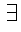#### 4.4.3.20 <abs/>

The abs element represents the absolute value of a real quantity or the modulus of a complex quantity.

The abs element takes the attribute definitionURL which can be used to override the default semantics.

The abs element is a unary arithmetic operator. See the Usage Guide for further details.

##### Example

The following example encodes the absolute value of x.


<apply>
<abs/>
<ci> x </ci>
</apply>

|x|

#### 4.4.3.21 <conjugate/>

The conjugate element represents the complex conjugate of a complex quantity.

The conjugate element takes the attribute definitionURL which can be used to override the default semantics.

The conjugate element is a unary arithmetic operator. See the Usage Guide for further details.

##### Example

The following example encodes the conjugate of x + i y.


<apply><conjugate/>
<apply><plus/>
<ci> x </ci>
<apply><times/>
<cn> &ImaginaryI; </cn>
<ci> y </ci>
</apply>
</apply>
</apply>

##### Default Rendering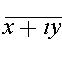### 4.4.4 Relations

#### 4.4.4.1 <eq/>

##### Discussion

The eq element is the "equals" relational operator.

The eq element takes the attribute definitionURL which can be used to override the default semantics.

The equals element is an n-ary relation. See the Usage Guide for further details.

##### Example
<reln><eq/>
<ci> a </ci>
<ci> b </ci>
</reln>

If this were tested at a = 5.5 and b = 6 it would yield the truth value "FALSE".
a = b

#### 4.4.4.2 <neq/>

##### Discussion

The neq element is the "not equal to" relational operator.

neq takes the attribute definitionURL which can be used to override the default semantics.

The neq element is an binary relation. See the Usage Guide for further details.

##### Example
<reln><neq/>
<ci> a </ci>
<ci> b </ci>
</reln>

If this were tested at a = 5.5 and b = 6 it would yield the truth value "TRUE".
##### Default Rendering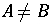#### 4.4.4.3 <gt/>

##### Discussion
The gt element is the "greater than" relational operator.

The gt element takes the attribute definitionURL which can be used to override the default semantics.

The gt element is an n-ary relation. See the Usage Guide for further details.

##### Example
<reln><gt/>
<ci> a </ci>
<ci> b </ci>
</reln>

If this were tested at a = 5.5 and b = 6 it would yield the truth value "FALSE".
A > B

#### 4.4.4.4 <lt/>

##### Discussion

The lt element is the "less than" relational operator.

The lt element takes the attribute definitionURL which can be used to override the default semantics.

The lt element is an n-ary relation. See the Usage Guide for further details.

##### Example
<reln><lt/>
<ci> a </ci>
<ci> b </ci>
</reln>

If this were tested at a = 5.5 and b = 6 it would yield the truth value "TRUE".
A < B

#### 4.4.4.5 <geq/>

##### Discussion

The geq element is the "greater than or equal" relational operator.

The geq element takes the attribute definitionURL which can be used to override the default semantics.

The geq element is an n-ary relation. See the Usage Guide for further details.

##### Example
<reln><geq/>
<ci> a </ci>
<ci> b </ci>
</reln>

If this were tested for a = 5.5 and b = 5.5 it would yield the truth value "TRUE".
##### Default Rendering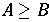#### 4.4.4.6 <leq/>

##### Discussion

The leq element is the "less than or equal" relational operator.

The leq element takes the attribute definitionURL which can be used to override the default semantics.

The leq element is an n-ary relation. See the Usage Guide for further details.

##### Example
<reln><leq/>
<ci> a </ci>
<ci> b </ci>
</reln>

If a = 5.4 and b = 5.5 this will yield the truth value "TRUE".
##### Default Rendering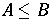### 4.4.5 Calculus

#### 4.4.5.1 <ln/>

##### Discussion

The ln element is the natural logarithm operator.

The ln element takes the attribute definitionURL which can be used to override the default semantics.

The ln element is an unary calculus operator. See the Usage Guide for further details.

##### Example
<apply><ln/>
<ci> a </ci>
</apply>

If a = e this will yield the value 1.
ln a

#### 4.4.5.2 <log/>

##### Discussion
The log element is the operator which returns a logarithm to a given base. The base may be specified using a logbase element, which should be the first child of the containing apply element. If the logbase element is not present, a default base of 10 is assumed.

The log element takes the attribute definitionURL which can be used to override the default semantics.

The log element can be used as either an operator taking qualifiers or a unary calculus operator. See the Usage Guide for further details.

##### Example
<apply> <log/>
<logbase>
<cn> 3 </cn>
</logbase>
<ci> x </ci>
</apply>

This markup represents "the base 3 log of x". For natural logarithms base e, the ln element should be used instead.
log3 x

#### 4.4.5.3 <int/>

##### Discussion

The int element is the operator element for an integral. The lower limit, upper limit and bound variable are given by (optional) child elements, lowlimit, uplimit and bvar in the enclosing apply element. The integrand is also specified as a child element of the enclosing apply element.

The domain of integration may alternatively be specified by using an interval element, or by a condition element. In such cases, if a bound variable of integration is intended, it must be specified explicitly. (The condition may involve more than one symbol.)

The int element takes the attribute definitionURL which can be used to override the default semantics.

The int element an operator taking qualifiers. See the Usage Guide for further details.

##### Examples

This example specifies a lowlimit, uplimit, and bvar.

<apply>
<int/>
<bvar>
<ci> x </ci>
</bvar>
<lowlimit>
<cn> 0 </cn>
</lowlimit>
<uplimit>
<ci> a </ci>
</uplimit>
<apply>
<fn><ci> f </ci></fn>
<ci> x </ci>
</apply>
</apply>


This example specifies the domain of integration with an interval element.

<apply><int/>
<bvar>
<ci> x </ci>
</bvar>
<interval>
<ci> a </ci>
<ci> b </ci>
</interval>
<apply><cos/>
<ci> x </ci>
</apply>
</apply>


The final example specifies the domain of integration with an condition element.

<apply><int/>
<bvar>
<ci> x </ci>
</bvar>
<condition>
<reln><in/>
<ci> x </ci>
<ci type="set"> D </ci>
</reln>
</condition>
<apply><fn><ci> f </ci></fn>
<ci> x </ci>
</apply>
</apply>

##### Default Rendering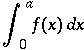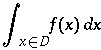#### 4.4.5.4 <diff/>

##### Discussion

The diff element is the differentiation operator element for functions of a single real variable. The bound variable is given by a bvar element which is a child of the containing apply element. The bvar elements may also contain a degree element, which specifies the order of the derivative to be taken.

The diff element takes the attribute definitionURL which can be used to override the default semantics.

The diff element is an operator taking qualifiers. See the Usage Guide for further details.

##### Example
<apply><diff/>
<bvar>
<ci> x </ci>
</bvar>
<apply><fn><ci> f </ci></fn>
<ci> x </ci>
</apply>
</apply>

##### Default Rendering#### 4.4.5.5 <partialdiff/>

##### Discussion

The partialdiff element is the partial differentiation operator element for functions of several real variables. The bound variables are given by bvar elements, which are children of the containing apply element. The bvar elements may also contain a degree element, which specifies the order of the partial derivative to be taken in that variable.

The partialdiff element takes the attribute definitionURL which can be used to override the default semantics.

The partialdiff element is an operator taking qualifiers. See the Usage Guide for further details.

##### Example
<apply><partialdiff/>
<bvar>
<ci> x </ci>
<degree>
<cn> 2 </cn>
</degree>
</bvar>
<bvar>
<ci> y </ci>
</bvar>
<apply><fn><ci> f </ci></fn>
<ci> x </ci>
<ci> y </ci>
</apply>
</apply>

##### Default Rendering#### 4.4.5.6 <lowlimit>

##### Discussion

The lowlimit element is the container element used to indicate the "lower limit" of an operator using qualifiers. For example, in an integral, it can be used to specify the lower limit of integration. Similarly, it is also used to specify the lower limit of an index for sums and products.

The meaning of the lowlimit element depends on the context it is being used in. For further details about how qualifiers are used in conjunction with operators taking qualifiers, consult the Usage Guide.

##### Example
<apply><int/>
<bvar>
<ci> x </ci>
</bvar>
<lowlimit>
<ci> a </ci>
</lowlimit>
<uplimit>
<ci> b </ci>
</uplimit>
<apply><fn><ci> f </ci></fn>
<ci> x </ci>
</apply>
</apply>


##### Default Rendering

The default rendering of the lowlimit element and its contents depends on the context. In the preceding example, it should be rendered as a subscript to the integral sign: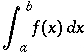Consult the descriptions of individual operators which make use of the lowlimit construct for default renderings.

#### 4.4.5.7 <uplimit>

##### Discussion

The uplimit element is the container element used to indicate the "upper limit" of an operator using qualifiers. For example, in an integral, it can be used to specify the upper limit of integration. Similarly, it is also used to specify the upper limit of an index for sums and products.

The meaning of the uplimit element depends on the context it is being used in. For further details about how qualifiers are used in conjunction with operators taking qualifiers, consult the Usage Guide.

##### Example
<apply><int/>
<bvar>
<ci> x </ci>
</bvar>
<lowlimit>
<ci> a </ci>
</lowlimit>
<uplimit>
<ci> b </ci>
</uplimit>
<apply><fn><ci> f </ci></fn>
<ci> x </ci>
</apply>
</apply>

##### Default Rendering

The default rendering of the uplimit element and its contents depends on the context. In the preceding example, it should be rendered as a superscript to the integral sign:Consult the descriptions of individual operators which make use of the uplimit construct for default renderings.

#### 4.4.5.8 <bvar>

##### Discussion
The bvar element is the container element for the "bound variable" of an operation. For example, in an integral it specifies the variable of integration. In a derivative, it indicates which variable with respect to which a function is being differentiated. When the bvar element is used to quantifiy a derivative, the bvar element may contain a child degree element which specifies the order of the derivative with respect to that variable. The bvar element is also used for the internal variable in sums and products and for the bound variable used with the universal and existential quantifiers forall and exists.

The meaning of the bvar element depends on the context it is being used in. For further details about how qualifiers are used in conjunction with operators taking qualifiers, consult the Usage Guide.

##### Examples
<apply><diff/>
<bvar>
<ci> x </ci>
<degree>
<cn> 2 </cn>
</degree>
</bvar>
<apply><power/>
<ci> x </ci>
<cn> 2 </cn>
</apply>
</apply>

<apply><int/>
<bvar><ci> x </ci></bvar>
<condition>
<reln><in/><ci> x </ci><ci> D </ci></reln>
</condition>
<apply><fn><ci> f </ci></fn>
<ci> x </ci>
</apply>
</apply>

##### Default Rendering

The default rendering of the bvar element and its contents depends on the context. In the preceding examples, it should be rendered as the x in the d x of the integral, and as the x in the denominator of the derivative symbol:Note that in the case of the derivative, the default rendering of the degree child of the bvar element is as an exponent.

Consult the descriptions of individual operators which make use of the uplimit construct for default renderings.

#### 4.4.5.9 <degree>

##### Discussion

The degree element is the container element for the "degree" or "order" of an operation. There are a number basic mathematical constructs which come in families, such as derivatives and moments. Rather than introduce special elements for each of these families, MathML uses a single general construct, the degree element for this concept of "order".

The meaning of the degree element depends on the context it is being used in. For further details about how qualifiers are used in conjunction with operators taking qualifiers, consult the Usage Guide.

##### Example
<apply><partialdiff/>
<bvar>
<ci> x </ci>
<degree>
<ci> n </ci>
</degree>
</bvar>
<bvar>
<ci> y </ci>
<degree>
<ci> m </ci>
</degree>
</bvar>
<apply><sin/>
<apply> <times/>
<ci> x </ci>
<ci> y </ci>
</apply>
</apply>
</apply>

##### Default Rendering

The default rendering of the degree element and its contents depends on the context. In the preceding example, the degree elements would be rendered as the exponents in the differentiation symbols: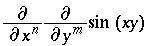Consult the descriptions of individual operators which make use of the uplimit construct for default renderings.

### 4.4.6 Theory of Sets

#### 4.4.6.1 <set>

##### Discussion

The set element is the container element which constructs a set of elements. The elements of a set can be defined either by explicitly listing the elements, or by using the bvar and condition elements.

The set element is a constructor container. See the Usage Guide for further details.

##### Examples
<set>
<ci> b </ci>
<ci> a </ci>
<ci> c </ci>
</set>

<set>
<bvar><ci> x </ci></bvar>
<condition>
<reln><lt/>
<ci> x </ci>
<cn> 5 </cn>
</reln>
</condition>
</set>

1. {a,b,c}
2. {x | x < 5 }

#### 4.4.6.2 <list>

##### Discussion

The list element is the container element which constructs a list of elements. Elements can be defined either by explicitly listing the elements, or by using the bvar and condition elements.

Lists differ from sets in that there is an explicit order to the elements. Two orders are supported: lexicographic and numeric. The kind of ordering which should be used is specified by the order attribute.

The list element is a constructor container. See the Usage Guide for further details.

##### Examples
<list>
<ci> b </ci>
<ci> a </ci>
<ci> c </ci>
</list>

<list order="numeric">
<bvar><ci> x </ci></bvar>
<condition>
<reln>
<ci> x </ci>
<cn> 5 </cn>
</reln>
</condition>
</list>

1. [a, b, c]
2. [x | x < 5 ]

#### 4.4.6.3 <union/>

##### Discussion

The union element is the operator for a set-theoretic union or join of two (or more) sets.

The union attribute takes the attribute definitionURL which can be used to override the default semantics.

The union element is an n-ary set operator. See the Usage Guide for further details.

##### Example
<apply><union/>
<ci> A </ci>
<ci> B </ci>
</apply>

##### Default Rendering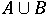#### 4.4.6.4 <intersect/>

##### Discussion
The intersect element is the operator for the set-theoretic intersection or meet of two (or more) sets.

The intersect element takes the attribute definitionURL which can be used to override the default semantics.

The intersect element is an n-ary set operator. See the Usage Guide for further details.

##### Example
<apply><intersect/>
<ci type="set"> A </ci>
<ci type="set"> B </ci>
</apply>

##### Default Rendering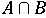#### 4.4.6.5 <in/>

##### Discussion

The in element is the relational operator used for a set-theoretic inclusion ("is in" or "is a member of").

The in element takes the attribute definitionURL which can be used to override the default semantics.

The in element is a binary set relation. See the Usage Guide for further details.

##### Example
<reln>
<in/>
<ci> a </ci>
<ci type="set"> A </ci>
</reln>

##### Default Rendering#### 4.4.6.6 <notin/>

##### Discussion
The notin element is the relational operator element used for set-theoretic exclusion ("is not in" or "is not a member of").

The notin element takes the attribute definitionURL which can be used to override the default semantics.

The notin element is a binary set relation. See the Usage Guide for further details.

##### Example
<reln><notin/>
<ci> a </ci>
<ci> A </ci>
</reln>

##### Default Rendering#### 4.4.6.7 <subset/>

##### Discussion

The subset element is the realtional operator element for a set-theoretic containment ("is a subset of").

The subset element takes the attribute definitionURL which can be used to override the default semantics.

The subset element is an n-ary set relation. See the Usage Guide for further details.

##### Example
<reln><subset/>
<ci> A </ci>
<ci> B </ci>
</reln>

##### Default Rendering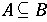#### 4.4.6.8 <prsubset/>

##### Discussion

The prsubset element is the relational operator element for set-theoretic proper containment ("is a proper subset of").

The prsubset element takes the attribute definitionURL which can be used to override the default semantics.

The subset element is an n-ary set relation. See the Usage Guide for further details.

##### Example
<reln><prsubset/>
<ci> A </ci>
<ci> B </ci>
</reln>

##### Default Rendering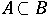#### 4.4.6.9 <notsubset/>

##### Discussion

The notsubset is the relational operator element for the set-theoretic relation "is not a subset of".

The notsubset element takes the attribute definitionURL which can be used to override the default semantics.

The notsubset element is a binary set relation. See the Usage Guide for further details.

##### Example
<reln><notsubset/>
<ci> A </ci>
<ci> B </ci>
</reln>

##### Default Rendering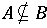#### 4.4.6.10 <notprsubset/>

##### Discussion

The notprsubset is the operator element for the set-theoretic relation "is not a proper subset of".

The notprsubset takes the attribute definitionURL which can be used to override the default semantics.

The notprsubset element is a binary set relation. See the Usage Guide for further details.

##### Example
<reln><notprsubset/>
<ci> A </ci>
<ci> B </ci>
</reln>

##### Default Rendering#### 4.4.6.11 <setdiff/>

##### Discussion

The setdiff is the operator element for a set-theoretic difference of two sets.

The setdiff element takes the attribute definitionURL which can be used to override the default semantics. setdiff is a binary operator.

The setdiff element is a binary set operator. See the Usage Guide for further details.

##### Example
<apply><setdiff/>
<ci> A </ci>
<ci> B </ci>
</apply>

##### Default Rendering### 4.4.7 Sequences and Series

#### 4.4.7.1 <sum/>

##### Discussion

The sum element denotes the summation operator. Upper and lower limits for the sum, and more generally a domains for the bound variables are specified using uplimit, lowlimit or a condition on the bound variables. The index for the summation is specified by a bvar element.

The sum element takes the attribute definitionURL which can be used to override the default semantics.

The sum element is an operator taking qualifiers. See the Usage Guide for further details.

##### Examples
<apply><sum/>
<bvar>
<ci> x </ci>
</bvar>
<lowlimit>
<ci> a </ci>
</lowlimit>
<uplimit>
<ci> b </ci>
</uplimit>
<apply><fn><ci> f </ci></fn>
<ci> x </ci>
</apply>
</apply>

<apply><sum/>
<bvar>
<ci> x </ci>
</bvar>
<condition>
<reln> <in/>
<ci> x </ci>
<ci type="set"> B </ci>
</reln>
</condition>
<apply><fn><ci> f </ci></fn>
<ci> x </ci>
</apply>
</apply>

##### Default Rendering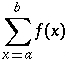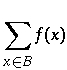#### 4.4.7.2 <product/>

##### Discussion

The product element denotes the product operator. Upper and lower limits for the product, and more generally a domains for the bound variables are specified using uplimit, lowlimit or a condition on the bound variables. The index for the product is specified by a bvar element.

The product element takes the attribute definitionURL which can be used to override the default semantics.

The product element is an operator taking qualifiers. See the Usage Guide for further details.

##### Examples
<apply><product/>
<bvar>
<ci> x </ci>
</bvar>
<lowlimit>
<ci> a </ci>
</lowlimit>
<uplimit>
<ci> b </ci>
</uplimit>
<apply><fn><ci> f </ci></fn>
<ci> x </ci>
</apply>
</apply>

<apply><product/>
<bvar>
<ci> x </ci>
</bvar>
<condition>
<reln> <in/>
<ci> x </ci>
<ci type="set"> B </ci>
</reln>
</condition>
<apply><fn><ci> f </ci></fn>
<ci> x </ci>
</apply>
</apply>

Usage Guide
##### Default Rendering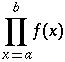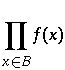#### 4.4.7.3 <limit/>

##### Discussion

The limit element is operation of taking a limit of a sequence. The limit point is expressed by specifying a lowlimit and a bvar or by specifying a condition on one or more bound variables.

The limit element takes the attribute definitionURL which can be used to override the default semantics.

The limit element is an operator taking qualifiers. See the Usage Guide for further details.

##### Examples
<apply><limit/>
<bvar>
<ci> x </ci>
</bvar>
<lowlimit>
<cn> 0 </cn>
</lowlimit>
<apply><sin/>
<ci> x </ci>
</apply>
</apply>

<apply><limit/>
<bvar>
<ci> x </ci>
</bvar>
<condition>
<reln>
<tendsto type="above"/>
<ci> x </ci>
<ci> a </ci>
</reln>
</condition>
<apply><sin/>
<ci> x </ci>
</apply>
</apply>

##### Default Rendering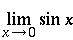#### 4.4.7.4 <tendsto/>

##### Discussion

The tendsto element is used to express the relation that a quantity is tending to a specified value.

The tendsto element takes the attributes type to set the direction from which the the limiting value is approached and definitionURL which can be used to override the default semantics.

The tendsto element is a binary relational operator. See the Usage Guide for further details.

##### Examples
<reln>
<tendsto type="above"/>
<apply><power/>
<ci> x </ci>
<cn> 2 </cn>
</apply>
<apply><power/>
<ci> a </ci>
<cn> 2 </cn>
</apply>
</reln>


To express (x, y) -> (f(x, y), g(x, y)), one might use vectors, as in:

<reln>
<tendsto/>
<vector>
<ci> x </ci>
<ci> y </ci>
</vector>
<vector>
<apply><fn><ci> f </ci></fn>
<ci> x </ci>
<ci> y </ci>
</apply>
<apply><fn><ci> g </ci></fn>
<ci> x </ci>
<ci> y </ci>
</apply>
</vector>
</reln>

##### Default Rendering
x2 -> a2
(x, y) -> (f(x, y), g(x, y))

### 4.4.8 Trigonometry

The names of the common trigonometric functions supported by MathML are listed below. Since their standard intepretations are widely known, they are discussed as a group.



##### Discussion

These operator elements denote the standard trigonometrical functions.

These elements all take the attribute definitionURL which can be used to override the default semantics.

They are all unary trigonometric operators. See the Usage Guide for further details.

##### Examples
<apply><sin/>
<ci> x </ci>
</apply>

<apply><sin/>
<apply><plus/>
<apply><cos/>
<ci> x </ci>
</apply>
<apply><power/>
<ci> x </ci>
<cn> 3 </cn>
</apply>
</apply>
</apply>

sin x
sin(cos x + x3)

### 4.4.9 Statistics

#### 4.4.9.1 <mean/>

##### Discussion
mean is the operator element for a mean or average.

mean takes the attribute definitionURL which can be used to override the default semantics.

##### Example
mean is an n-ary operator.
<apply><mean/>
<ci> X </ci>
</apply>

Usage Guide
##### Default Rendering#### 4.4.9.2 <sdev/>

##### Discussion
sdev is the operator element for the standard deviation.

sdev takes the attribute definitionURL which can be used to override the default semantics.

##### Example
sdev is an n-ary operator.
<apply><sdev/>
<ci> X </ci>
</apply>

Usage Guide
##### Default Rendering#### 4.4.9.3 <variance/>

##### Discussion
variance is the operator element for the statistical variance.

variance takes the attribute definitionURL which can be used to override the default semantics.

##### Example
variance is an n-ary operator.
<apply><variance/>
<ci> X </ci>
</apply>

Usage Guide
##### Default Rendering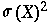#### 4.4.9.4 <median/>

##### Discussion
median is the operator element for the median .

median takes the attribute definitionURL which can be used to override the default semantics.

##### Example
median is an n-ary operator.
<apply><median/>
<ci> X </ci>
</apply>

Usage Guide
median(X)

#### 4.4.9.5 <mode/>

##### Discussion
mode is the operator for the statistical mode.

mode takes the attribute definitionURL which can be used to override the default semantics.

##### Example
mode is an n-ary operator.
<apply>
<mode/>
<ci> X </ci>
</apply>

Usage Guide
mode(X)

#### 4.4.9.6 <moment/>

##### Discussion
The moment element represents statistical moments. Use degree for the n in "nth moment".

moment takes the attribute definitionURL which can be used to override the default semantics.

##### Example
moment is an operator taking qualifiers.
<apply><moment/>
<degree>
<cn> 3 </cn>
</degree>
<ci> X </ci>
</apply>

Usage Guide
##### Default Rendering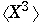### 4.4.10 Linear Algebra

#### 4.4.10.1 <vector>

##### Discussion
vector is the container element for a vector. The child elements form the components of the vector.

For purposes of interaction with matrices and matrix multiplication vectors are regarded as equivalent to a matrix consisting of a single column and the transpose of a vector behaves the same as a matrix consisting of a single row.

##### Example
vector is a constructor element.
<vector>
<cn> 1 </cn>
<cn> 2 </cn>
<cn> 3 </cn>
<ci> x </ci>
</vector>

Usage Guide
##### Default Rendering#### 4.4.10.2 <matrix>

##### Discussion
The matrix is the container element for matrixrow's. matrixrow's contain the elements of a matrix.
##### Example
matrix is a constructor element.
<matrix>
<matrixrow>
<cn> 0 </cn> <cn> 1 </cn> <cn> 0 </cn>
</matrixrow>
<matrixrow>
<cn> 0 </cn> <cn> 0 </cn> <cn> 1 </cn>
</matrixrow>
<matrixrow>
<cn> 1 </cn> <cn> 0 </cn> <cn> 0 </cn>
</matrixrow>
</matrix>

Usage Guide
##### Default Rendering#### 4.4.10.3 <matrixrow>

##### Discussion
The matrixrow element is the container element for the rows of a matrix.
##### Example
matrixrow is a constructor element.
<matrixrow>
<cn> 1 </cn>
<cn> 2 </cn>
</matrixrow>
<matrixrow>
<cn> 3 </cn>
<ci> x </ci>
</matrixrow>

Usage Guide
##### Default Rendering
Matrix rows are not directly rendered by themselves outside of the context of a matrix.

#### 4.4.10.4 <determinant/>

##### Discussion
The determinant element is the operator for constructing the determinant of a matrix.

determinant takes the attribute definitionURL which can be used to override the default semantics.

##### Example
determinant is an unary operator.
<apply><determinant/>
<ci type="matrix"> A </ci>
</apply>

Usage Guide
det A

#### 4.4.10.5 <transpose/>

##### Discussion
The transpose element is the operator for constructing the transpose of a matrix.

transpose takes the attribute definitionURL which can be used to override the default semantics.

##### Example
transpose is an unary operator.
<apply><transpose/>
<ci type="matrix"> A </ci>
</apply>

Usage Guide
At

#### 4.4.10.6 <selector/>

##### Discussion

The selector element is the operator for indexing into vectors matrices and lists. It accepts one or more arguments. The first argument identifies the vector, matrix or list from which the selection is taking place, and the second and subsequent arguments, if any, indicate the kind of selection taking place.

When selector is used with a single argument, it should be interpreted as giving the sequence of all elements in the list, vector or matrix given. The ordering of elements in the sequence for a matrix is understood to be first by column, then by row. That is, for a matrix (ai,j), where the indicies denote row and column, the ordering would be a1,1, a1,2, ... a2,1, a2,2 ... etc

When three arguments are given, the last one is ignored for a list or vector, and in the case of a matrix, the second and third arguments specify the row and column of the selected element.

When two arguments are given, and the first is a vector or list, the second argument specifies an element in the list or vector. When a matrix and only one index i is specified as in

<apply><selector/>
<matrix>
<matrixrow>
<cn> 1 </cn> <cn> 2 </cn>
</matrixrow>
<matrixrow>
<cn> 3 </cn> <cn> 4 </cn>
</matrixrow>
</matrix>
<cn> 1 </cn>
</apply>

it refers to ith matrixrow. Thus, the preceding example selects the following row:
<matrixrow> <cn> 1 </cn> <cn> 2 </cn> </matrixrow>


selector takes the attribute definitionURL which can be used to override the default semantics.

selector is classified as an n-ary linear algebra operator even though it can take only one, two, or three arguments.

##### Example
<apply><selector/>
<ci type="matrix"> A </ci>
<cn> 3 </cn>
<cn> 2 </cn>
</apply>

##### Default Rendering
The selector construct renders the same as the expression which is selects.

### 4.4.11 Semantic Mapping Elements

The use of the semantic mapping elements is explained in section 4.4.6

#### 4.4.11.1 <annotation>

##### Discussion
The annotation element is the container element for a semantic annotation in a non-XML format.

annotation takes the attribute encoding to define the encoding being used.

##### Example
annotation is a semantic mapping element. It is always used with semantics.
<semantics>

<apply><plus/>
<apply><sin/>
<ci> x </ci>
</apply>
<cn> 5 </cn>
</apply>

<annotation encoding="TeX">
\sin x + 5
</annotation>

</semantics>

##### Default Rendering
None. The information contained in annotations may optionally be used by a renderer able to process the kind of annotation given.

#### 4.4.11.2 <semantics>

##### Discussion
The semantics element is the container element which associates additional representations with a given MathML construct. The semantics element has as its first child the expression being annotated, and the subsequent children are the annotations. There is no restriction on the kind of annotation that can be attached using the semantics element. For example, one might give a TeX encoding, or computer algebra input in an annotation.

The representations which are XML based are enclosed in an annotation-xml element while those representations which are to be parsed as PCDATA are enclosed in an annotation element.

semantics takes the attribute definitionURL which can be used to reference an external source for some or all of the semantic information.

An important purpose of the semantics construct is to associate specific semantics with a particular presentation, or additional presentation information with a content construct. The default rendering of a semantics element is the default rendering of its first child. When a MathML-presentation annotation is provided, a MathML renderer may optionally use this information to render the MathML construct. This would typically be the case when the first child is a MathML content construct and the annotation is provided to give a preferred rendering differing from the default for the content elements.

##### Examples and Usage
semantics is a semantic mapping element.
<semantics>
<apply><plus/>
<apply><sin/>
<ci> x </ci>
</apply>
<cn> 5 </cn>
</apply>

<annotation encoding="Maple">
sin(x) + 5
</annotation>

<annotation-xml encoding="MathML-Presentation">
...
...
</annotation-xml>

<annotation encoding="Mathematica">
Sin[x] + 5
</annotation>

<annotation encoding="TeX">
\sin x + 5
</annotation>

<annotation-xml encoding="OpenMath">
<OMA>..</OMA>
</annotation-xml>

</semantics>

##### Default Rendering
The default rendering of a semantics element is the default rendering of its first child.

#### 4.4.11.3 <annotation-xml>

##### Discussion
The annotation-xml container element is used to contain representations that are XML based. It is always used together with the semantics element, and takes the attribute encoding to define the encoding being used.

annotation-xml is a semantic mapping element.

##### Example
<semantics>

<apply><plus/>
<apply><sin/>
<ci> x </ci>
</apply>
<cn> 5 </cn>
</apply>

<annotation-xml encoding="OpenMath">
<OMA><OMS name="plus"/>
<OMA><OMS name="sin"/>
<OMV name="x"/>
</OMA>
5
</OMA>
</annotation-xml>

</semantics>